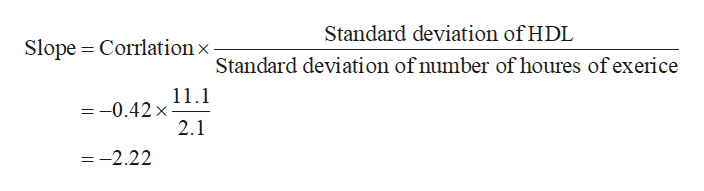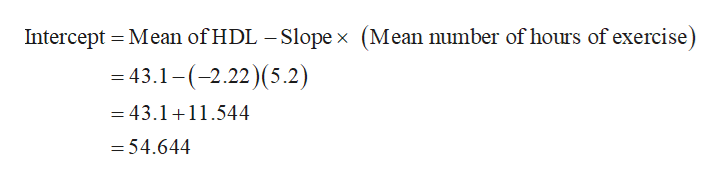# Consider the data below. Suppose that in the aerobic exercise group we also measured the number of hours of aerobic exercise per week; the mean is 5.2 hours with a standard deviation of 2.1 hours. The sample correlation is -0.42. a) Estimate the equation of the regression line that best describes the relationship between number of hours of exercise per week and HDL cholesterol level (Assume that the dependent variable is HDL level). b) Estimate the HDL level for a person who exercises 7 hours per week. c) Estimate the HDL level for a person who does not exercise.Exercise GroupNMeanStandard DeviationWeight Training 2049.710.2Aerobic Exercise 2043.111.1Stretching/Yoga2057.012.5

Question
127 views

Consider the data below. Suppose that in the aerobic exercise group we also measured the number of hours of aerobic exercise per week; the mean is 5.2 hours with a standard deviation of 2.1 hours. The sample correlation is -0.42. a) Estimate the equation of the regression line that best describes the relationship between number of hours of exercise per week and HDL cholesterol level (Assume that the dependent variable is HDL level). b) Estimate the HDL level for a person who exercises 7 hours per week. c) Estimate the HDL level for a person who does not exercise.

 Exercise Group N Mean Standard Deviation Weight Training 20 49.7 10.2 Aerobic Exercise 20 43.1 11.1 Stretching/Yoga 20 57.0 12.5
check_circle

Step 1

It is given that the sample correlation is –0.42.

Step 2

a.

The slope of the regression equation is obtained as follows:help_outlineImage TranscriptioncloseStandard deviation of HDL Slope Corrlation x Standard deviation of number of houres of exerice 11.1 =-0.42 x 2.1 =-2.22 fullscreen
Step 3

The intercept is obtained as follows:

...help_outlineImage TranscriptioncloseIntercept Mean of HDL - Slope x (Mean number of hours of exercise) -43.1-(2.22)(5.2) - 43.1 +11.544 =54.644 fullscreen

### Want to see the full answer?

See Solution

#### Want to see this answer and more?

Solutions are written by subject experts who are available 24/7. Questions are typically answered within 1 hour.*

See Solution
*Response times may vary by subject and question.
Tagged in

### Statistics